# Linear Programming: Simplex with 3 Decision Variables

## The Linear Programming Problem

Solve this linear programming problem.

 Maximize Subject to: P = 20x1 + 10x2 + 15x3 3x1 + 2x2 + 5x3 ≤ 55 2x1 + x2 + x3 ≤ 26 x1 + x2 + 3x3 ≤ 30 5x1 + 2x2 + 4x3 ≤ 57 x1 , x2 , x3 ≥ 0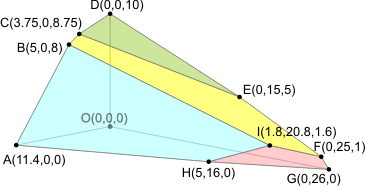The feasible region is the solid bounded by the planes shown in the figure.

This also demonstrates why we don't try to graph the feasible region when there are more than two decision variables. Three dimensional graphs aren't that easy to draw and you can forget about making the sketch when there are four or more decision variables.

The table method doesn't work that well either. Each intersection point is the the solution to a 3×3 system of linear equations. There are 7C3 = 35 intersection points for a problem this size, yet only ten of them are corner points in this case.

## The Initial System

The initial system is found by converting the ≤ constraints into = constraints by adding a slack variable.

 Maximize Subject to: P = 20x1 + 10x2 + 15x3 3x1 + 2x2 + 5x3 + s1 = 55 2x1 + x2 + x3 + s2 = 26 x1 + x2 + 3x3 + s3 = 30 5x1 + 2x2 + 4x3 + s4 = 57 x1 , x2 , x3 , s1 , s2 , s3 , s4 ≥ 0

In three dimensions, the inequalities become planes rather than lines like they were in two dimensions. Each plane can be uniquely identified by setting one of the variables equal to zero. The relationship between the variables and the planes is shown in the figure below. We'll refer back to this figure as we work through the Simplex procedure.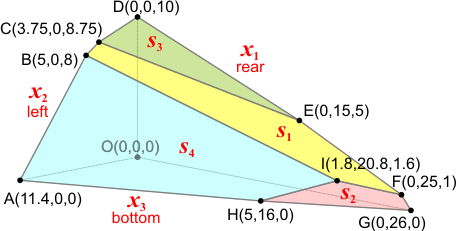## The Initial Tableau

Convert the system into a tableau. Remember to move all of the objective function terms to the left side and place it in the last row of the tableau.

x1 x2 x3 s1 s2 s3 s4 P rhs
3 2 5 1 0 0 0 0 55
2 1 1 0 1 0 0 0 26
1 1 3 0 0 1 0 0 30
5 2 4 0 0 0 1 0 57
-20 -10 -15 0 0 0 0 1 0

### Interpreting the Tableau

Determine which variable is basic in each row. The cleared columns correspond to basic variables and their variable is the basic variable for the row in which they have their single value. Columns that are not cleared (have more than one non-zero value in them) are non-basic and are zero.

Basic   x1 x2 x3 s1 s2 s3 s4 P rhs
s1   3 2 5 1 0 0 0 0 55
s2   2 1 1 0 1 0 0 0 26
s3   1 1 3 0 0 1 0 0 30
s4   5 2 4 0 0 0 1 0 57
P   -20 -10 -15 0 0 0 0 1 0

Here are the values of the variables for this tableau.

Basic Non-Basic
s1=55, s2=26, s3=30, s4=57, P=0 x1=0, x2=0, x3=0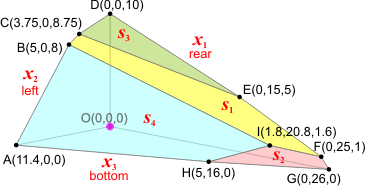This tableau corresponds to point O (0,0,0) of the feasible region. Notice that point O is at the intersection of the three planes corresponding to the non-basic variables: x1=0 (rear), x2=0 (left), and x3=0 (bottom).

### Determining the Pivot

Since there are still negatives in the bottom row, we're not done. Pick the column that has the most negative value in the bottom row and that is your pivot column. Then find the ratios between the non-negative values on the right hand side and the positive values in the pivot column. The smallest non-negative ratio is the pivot row.

Basic   x1 x2 x3 s1 s2 s3 s4 P rhs   ratio
s1   3 2 5 1 0 0 0 0 55   55/3 = 18.3
s2   2 1 1 0 1 0 0 0 26   26/2 = 13.0
s3   1 1 3 0 0 1 0 0 30   30/1 = 30.0
s4   5 2 4 0 0 0 1 0 57   57/5 = 11.4
P   -20 -10 -15 0 0 0 0 1 0

In this case, we'll pivot on Row 4, Column 1. x1 will be entering the set of basic variables and replacing s4, which is exiting. We also know that the increase in the objective function will be 20×11.4 = 228.

## Second Tableau

I've gone ahead and labeled the tableau with the pivot column, ratios, and pivot row. This was done just to save time.

Basic   x1 x2 x3 s1 s2 s3 s4 P rhs   ratio
s1   0 0.8 2.6 1 0 0 -0.6 0 20.8   26
s2   0 0.2 -0.6 0 1 0 -0.4 0 3.2   16
s3   0 0.6 2.2 0 0 1 -0.2 0 18.6   31
x1   1 0.4 0.8 0 0 0 0.2 0 11.4   28.5
P   0 -2 1 0 0 0 1 1 228

Here are the values of the variables for this tableau.

Basic Non-Basic
x1=11.4, s1=20.8, s2=3.2, s3=18.6, P=169 x2=0, x3=0, s4=0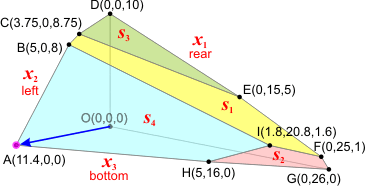This corresponds to point A (11.4,0,0). Notice that point A is the intersection of the three planes x2=0 (left), x3=0 (bottom), s4=0 (cyan). Those are your non-basic variables.

In this case, we'll pivot on Row 2, Column 2. x2 will be entering the set of basic variables and replacing s2, which is exiting. We also know that the increase in the objective function will be 2×16 = 32. Since the value of the objective function is 228 right now, it will be 228+32=260 after the next pivot.

## Third Tableau

Basic   x1 x2 x3 s1 s2 s3 s4 P rhs   ratio
s1   0 0 5 1 -4 0 1 0 8   1.6
x2   0 1 -3 0 5 0 -2 0 16   na
s3   0 0 4 0 -3 1 1 0 9   2.25
x1   1 0 2 0 -2 0 1 0 5   2.5
P   0 0 -5 0 10 0 0 1 260

Notice that we can't find the ratio for the second row.

Here are the values of the variables for this tableau.

Basic Non-Basic
x1=5, x2=16, s1=8, s3=9, P=260 x3=0, s2=0, s4=0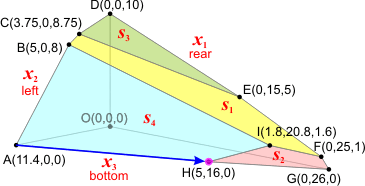This tableau corresponds to point H (5,16,0). Notice that point H is the intersection of the three planes x3=0 (bottom), s2=0 (pink), and s4=0 (cyan). Those are your non-basic variables.

Pivot on Row 1, Column 3. x3 will be entering the set of basic variables and replacing s1, which is exiting. The increase in the objective function will be 5×1.6 = 8, which make the objective function value be 260+8 = 268 after this pivot.

## Final Tableau

Basic   x1 x2 x3 s1 s2 s3 s4 P rhs
x3   0 0 1 0.2 -0.8 0 0.2 0 1.6
x2   0 1 0 0.6 2.6 0 -1.4 0 20.8
s3   0 0 0 -0.8 0.2 1 0.2 0 2.6
x1   1 0 0 -0.4 -0.4 0 0.6 0 1.8
P   0 0 0 1 6 0 1 1 268

This is the final tableau because there are no negatives in the bottom row.

Here are the values of the variables for this tableau.

Basic Non-Basic
x1=1.8, x2=20.8, x3=1.6, s3=2.6, P=268 s1=0, s2=0, s4=0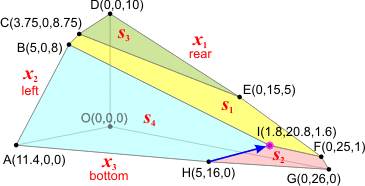This tableau corresponds to point I (1.8,20.8,1.6). Notice that point Iis the intersection of the three planes s1=0 (yellow), s2=0 (pink), and s4=0 (cyan). Those are your non-basic variables.

## We're Done!

Since there are no negatives in the bottom row, moving to another point would lower the value of the objective function, not raise it. Since we're trying to maximize the value of the objective function, that would be counter-productive. We stop, we're done.

The maximum value of P is 268 when x1 = 1.8, x2 = 20.8, and x3 = 1.6. The values of the slack variables are s1 = 0, s2 = 0, s3 = 2.6, and s4 = 0.